P18.5

A Theoretical and Numerical Study of Density Currents

in Non-constant Shear Flows

*Ming Xue, **Qin Xu and †**Kelvin K. Droegemeier

*Center for Analysis and Prediction of Storms

**Cooperative Institute for Mesoscale Meteorological Studies

†School of Meteorology

University of Oklahoma, Norman, Oklahoma 73019

1. Introduction

It is commonly accepted that the interaction between the environmental shear and the cold pool of a thunderstorm outflow (density current) may play an important role in producing long-lived squall lines (e.g. Thorpe, at al. 1982, TMM82; Rotunno et al. 1988, RKW88). To improve our understanding of the interaction between density currents and their environment, simple nonlinear, two-fluid steady-state models were recently developed by Xu (1992, X92) and Xu and Moncrieff (1994, XM94). Compared to the classic density current theory of Benjamin (1968), these models include, among other new ingredients, the effect of environmental shear. In Xu et al. (1996, XXD96), time-dependent numerical simulations were performed to validate the theoretical solutions of X92 and XM94. The numerical model was able to reproduce with high accuracy quasi-steady-state density currents in uniform flow and constant shear.

In this paper, we extend the theoretical models of X92 to a case where the inflow shear is confined to the lower part of a vertically bounded channel. The inflow above the shear layer is uniform. This flow configuration is illustrated in Fig. 1 and is of more relevance to meteorological applications since most long-lived convective systems occur in environments with most of the shear confined to the lowest a few kilometers of the tropopause (e.g. Bluestein and Jain, 1985).

In the following sections, we present first the theoretical model, then describe the design and results of numerical experiments and finally some conclusions in the last section.

2. The theoretical model

Similar to X92 and XM94, we examine an idealized steady-state model of density current moving at a constant speed in an environmental flow with low-level shear. (Fig.1). The variables of this model are non-dimensionalized using the following scaling:(2.1)

where the variables on the left hand side of the arrows are dimensional. Here, H is the depth of the domain bounded by two rigid boundaries;is the velocity scale; g is the acceleration of gravity;is the density difference across the cold pool interface andthe perturbation pressure. Here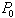is the unperturbed reference pressure in the upstream inflow associated with constant density.Figure 1. Schema of the steady-state model of a density current circulation in an environment with low-level shear.

In a framework moving with the density current front, the steady-state flow in Fig.1 can be fully described by a set of six non-dimensional parameters:(2.2)

where a is the low-level shear in the upstream inflow,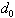the depth of this shear layer and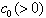the constant speed of inflow above the shear layer.is the depth of the shear layer immediately above the cold pool, and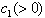is the speed of outflow at the cold pool top. Finally, h (0 < h < 1) is the depth of density current. According to vorticity conservation, the vorticity in the inflow and outflow shear layers should be the same therefore there is no need for another shear parameter.

Following Benjamin (1968), the remote system-relative environmental inflow and outflow are constrained by mass continuity, energy conservation and flow force balance. It can be shown that only two of the six parameters in (2.2) are independent. In this paper, we choose the depth () and vorticity (a) of the inflow shear as the independent control parameters.

According to Fig.1, the inflow profile is given by(2.3)

and the outflow profile is given by(2.4)

The mass continuity requires that the inflow mass flux be equal to the outflow mass flux for both shear and zero-shear layers. From these two conditions, we can obtain the following two constraints:(2.5)

and(2.6)

Furthermore, for a steady-state flow, the Bernoulli energy () is constant along a streamline. Apply this theorem along the interfacial streamline from the frontal nose B to the downstream point D, we obtain. Inside the cold pool, the flow speed is zero, therefore. Integrating the hydrostatic relation for the denser fluid inside the cold pool gives. Equating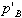andresults in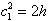, (2.7)

which is another constraint among the control parameters.

By integrating the steady-state horizontal momentum equation over the entire domain and making use of the mass continuity, one can obtain the flow force balance (see Benjamin 1968):.

Substituting velocity and pressure profiles into above, we obtain after certain algebra the fourth constraint:Now that we have four constraints as given in (2.5-8) for six control parameters, only two of them are free parameters. These four equations are solved numerically given values of a and. Solution h is plotted against a for differentin Fig.2. It can be seen that for=1, the solution reduces to that of X92. When a=0, the model degenerates to the classic density current solution (h = 0.5) of Benjamin (1968).

The density current becomes deeper (Fig.2) and propagates faster (figure not shown) relative to the surface inflow as the shear increases from negative through positive values. The positive shear raises the density current head above the 0.5 value of constant flow case while the negative shear contributes in an opposite direction. Correspondingly, the deeper density current propagates faster than the shallower ones. The effect of shear is more dramatic for a deep inflow shear and the solution tends to the limit of constant shear case of X92 whenapproaches 1 for relatively small values of a.Figure 2. The cold pool depth h plotted against inflow shear a for different values of shear depth, according to the steady-state theoretical model.

The flow structure near the front is also important. von Karman (1940) and Benjamin (1968) showed analytically that the frontal slope at the stagnation point is 60o for an idealized inviscid density current in a uniform environmental inflow with a free-slip boundary condition. X92 and XM94 further showed that this 60o angle is independent of the inflow shear and cold-pool circulation. They also showed that the mid-depth interface slope can exceed 60o for strong inflow shear. These findings can be extended to our case of non-constant vertical shear. In the next section, numerical experiments are performed to validate these theoretical results.

3. The numerical experiments

The Advanced Regional Prediction System (Xue et al. 1995) in used in its simplest 2-D setting with minimum physics. A number of approximations, including Boussinesq approximation, are made to the model equations, which are then non-dimensionalized.

A 40¥1 non-dimensional computational domain is used, and the density current is generated by placing an initially static block of cold air (b=-1) in the middle portion of the computational domain with a length of 8 at its top. The analytically specified interface of this block has a 60° slope at both ends. The domain is represented by 801¥41 grid points and all experiments are carried out to a non-dimensional time T=36.

The initial flow at the far upstream and downstream boundaries are specified according to Eq.(2.3). The interior flow is obtained numerically by solving a Possion equation for streamfunction based on mass and vorticity conservation. A similar procedure is used in XXD96.

A set of six experiments were conducted and their initial and simulated parameter values are listed in Table 1. Limited by space we will only discuss results from selected experiments.

Table 1. Initial settings and simulated values of the kinematic parameters of the numerical experiments.

 Model Specified Theoretical / Model Simulated a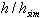LS1 1.0 0.2 0.59 0.590/0.538 0.412/0.400 LS2 -1.0 0.2 0.410 0.410/0.325 0.318/0.318 LS1A 1.0 0.2 0.410 0.590/0.531 0.412/0.412 DS 1.0 0.2 0.683 0.683/0.638 0.696/0.709 SLS 3.0 0.2 0.767 0.767/0.763 0.777/0.789 SLSA 3.0 0.2 0.200 0.767/0.763 0.777/0.635

In LS1 and LS2, the inflow shear depth d0=0.2 and the shear is moderately strong (|a|=1). They differ only in the sign of shear. The initial cold pool height (h0) is taken from Fig.2 (h0=0.59 and 0.41 respectively) therefore the initial cold pool is in a steady-state balance with the inflow profile. We expect that the density current front on the upstream side remain quasi-stationary and the current head maintain its initial height in the simulations.

In fact, between T=12 and 18, the frontal nose in LS1 only retreated by 0.075, yielding a flow relative propagation speed of 0.40, corresponding to a mere 3% error compared to the theoretical value (see Table 1). Measured by the -0.5 buoyancy contours in the time averaged field (Fig.3), the height of the simulated current head is about 0.538, only slightly shallower than the theoretical value of 0.59. It is worth noting that, due to Kelvin-Helmholtz eddies, the time averaged density current shows a noticeable decrease in its depth to the rear of its head. This resembles the hydraulic jump during which the flow transitions from a supercritical to a subcritical state. This process was discussed in X92. Note also that the slope of the front at the nose is very close to 60Ú.Figure 3. Non-dimensional velocity and buoyancy fields averaged over a period from T=12 to 18 for experiment LS1. The x origin indicates the initial position of the frontal nose. The areas with b"d-0.5 are shaded. The figure is plotted to the physical scale

A similar agreement with the theory is found for LS2. Between T=12 and 18, the frontal nose remained exactly stationary. The simulated head height is 0.325 (Table 1) versus the theoretical value of 0.410. The accuracy in the depth prediction is not as good as the positive shear (deeper density current) case. A similar behavior is found in XXD96 for uniform shear cases.

To test the dependency of the final behavior of simulated density current on the initial setting, we repeated experiment LS1 with h0=0.41. Interestingly, the initially lower current head is quickly raised to a level similar to that in LS1 by T=6. The front remain almost completely stationary between T=6 and 24 and the time averaged shape of the current head (figure not shown) appears very similar to Fig.3 of LS1, indicating the robustness of the numerical solutions.Figure 4. As Fig.3, but for experiment SLS.

Experiment SLS represents a case with a strong low level shear (a=3.0, d0=0.2). This configuration is expected to support a deep cold pool (h=0.767) and a mid-level frontal slope exceeding 60° (XM94). The slope of the mid-level frontal surface and the associated flow is particularly important since it can determine the longevity of squall line type convective system by modulating the updraft orientation (TMM82; RKW88).

Indeed, the simulated density current has a interface slope of more than 60° at the mid-levels (Fig.4). The depth of the current head and its propagation agree very well with the theoretical results (Table 1).

SLS has shown that deep density current is supported by strong low-level shear. In the atmosphere, where the troposphere (which is analogous to the model top lid) is usually about 10 km deep, the density currents forming out of thunderstorm outflow rarely exceeds 2 km in depth. It is of more practical importance in understanding the behavior of this type of density current. Towards this end, SLSA is performed with everything the same as SLS except that h0 is set to 0.2, much below the theoretical solution of 0.767. In this case, the given initial cold pool obviously can not resist the inflow, nor does it have enough mass to achieve the deep balanced depth. As a result, the density current recedes quickly. Between T=6 and 24, the front propagates at an average speed of -0.142, resulting a flow-relative speed of 0.635 (Table 1).

The flow at the density current head is highly unsteady. However, the time fields still exhibit an well organized flow pattern. The streamline field (not shown) depicts a predominant jump-type flow, and the buoyancy field (Fig.5) shows an envelope of the denser fluid extending to a height that is close to the theoretical head depth of 0.767. The shape of this envelope is not very different from that of the density current head in SLS. The results suggest that the basic dynamic control (pressure balance) on the flow interface is still working, despite the instantaneous flow is highly transient. This further confirms the role of the shear in the low-level inflow; positive shear in the low-level inflow does possess a power of strong vertical lifting at the frontal nose and stronger positive shear supports a deeper jump type or even an overturning flow.Figure 5. As Fig.3, but for experiment SLS1.

4. Summary and Conclusions

The earlier two-fluid idealized model of density current models have been extended to include non-constant vertical shear. It is found that the shear confined to the low levels plays a similar role as the uniform vertical shear in controlling the depth of density currents. Time-dependent numerical experiments are conducted that supports the theoretical model solution and confirms the role of low-level inflow shear in maintaining a deep convective updraft. More details will be reported in a journal article.

Acknowledgments. This research was supported by NOAA Grant NA37RJ0203 and NSF Grant ATM-9417304 to CIMMS, by NSF Grant ATM91-20009 to CAPS, and by NSF Grant ATM92-22576 to the third author.

REFERENCES

Benjamin, B.T., 1968. J. Fluid Mech., 31, 209-248.

Bluestein H.B. and M.H. Jain, 1985. J. Atmos. Sci. 42, 1711-1732.

Karman, T. von, 1940. Bull. Am. Math. Soc., 46, 615-683.

Rotunno, R., J.B. Klemp, and M.L. Weisman, 1988 (RKW88). J. Atmos. Sci., 45, 463-485.

Thorpe A.J., M.J. Miller and M.W. Moncrieff, 1982 (TMM82). Quart. J. Roy. Meteor. Soc., 108, 739-762.

Xu, Q., 1992 (X92). J. Atmos. Sci., 49, 511-524.

_____, and M. W. Moncrieff, 1994 (XM94). J. Atmos. Sci., 51, 434-446.

_____, Xue, M., and K.K. Droegemeier, 1996 (XXD96). J. Atmos. Sci., 53, 770-786.

Xue, M., K.K. Droegemeier, V. Wong, A. Shapiro, K. Brewster, 1995: Advanced Regional Prediction System (ARPS) Version 4.0 User's Guide, 380pp (Available from CAPS, Univer. of Oklahoma).